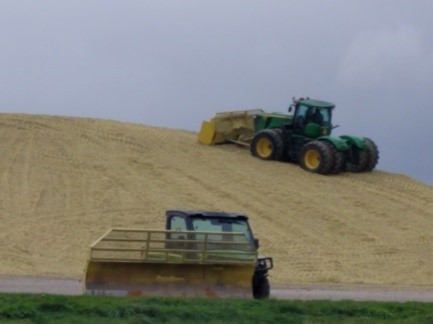# Determining the Tons of Silage in Piles for CFAPKevin Jarek, Liz Binversie, Bill Halfman, Paul Mitchell

This tool helps a farmer calculate the tons of silage in bunker silos and convert it to its grain equivalent for including in 2019 production for determining CFAP payments.

Step 1: Enter the various pile measurements in the yellow cells.

Step 2: In red, the Calculated Weight Per Pile is reported in pounds and to the right as tons dry matter (T DM).

Step 3: Divide Tons of Dry Matter by 0.35 to convert silage tons of DM to 65% moisture.

Step 4: Multiply by the FSA conversion factor. Corn silage is 7.94 bu/ton (at 65% moisture) and 4.08 bu/ton (at 65% moisture) for oatlage.

Example: Determine the wet tons of 65% silage in a partially fed silage pile.

1. Pile bottom length (without ramps) is 60 ft, the filing ramp is gone, so enter 0.01. The back ramp is 50 ft, the bottom width is 120 ft, and the pile depth is 10 ft. The dome height is 3 ft and the top width of the dome is 24 ft. Use the standard 15 lbs/ft3 dry matter density and 65% moisture.
2. The spreadsheet calculates 508 tons of dry matter weight in red.
3. Divide by 0.35 to convert to standard 65% moisture: 508/0.35 = 1,451 tons wet silage.
4. Convert to grain equivalents using FSA conversion factors:
• Corn silage: 1,451 x 7.94 bu/ton = 11,521 bu of Corn
• Oatlage: 1,451 x 4.08 bu/ton = 5,920 bu of Oats
• Soybean Silage: 1,451 x 5.00 bu/ton = 7,255 bu of Soybeans
• Grain Sorghum Silage 1,451 x 5.56 bu/ton = 8,068 bu of Sorghum